Anzeige

# Binary search tree

8. Mar 2018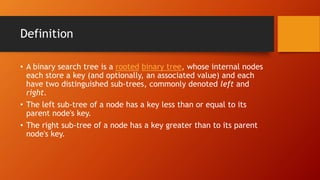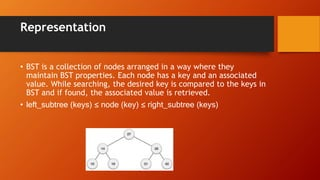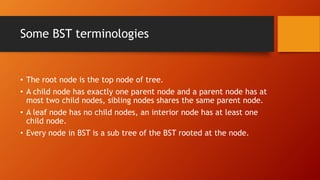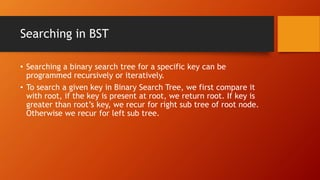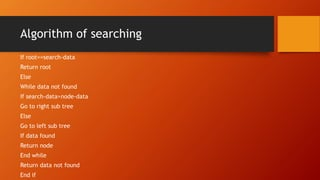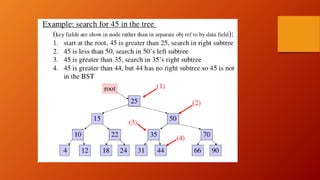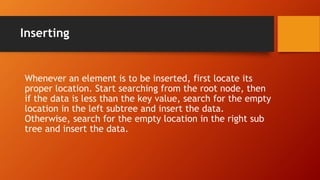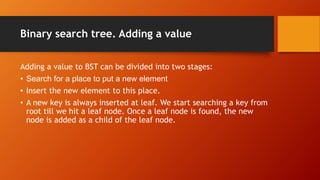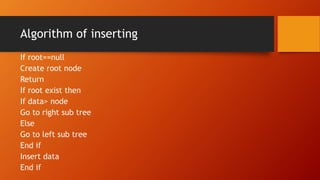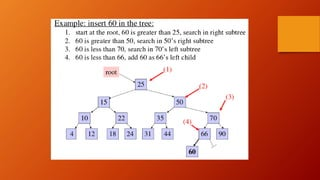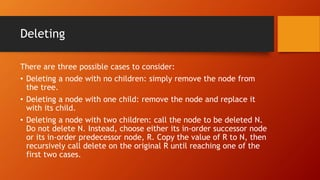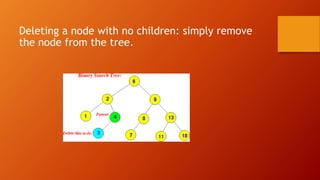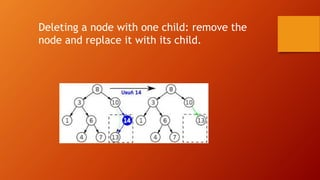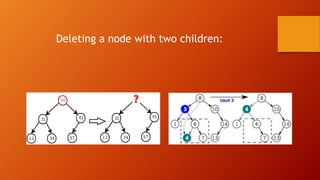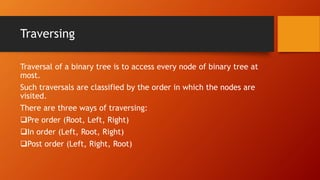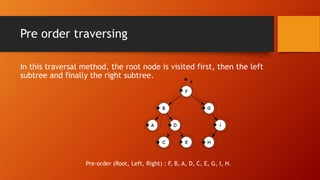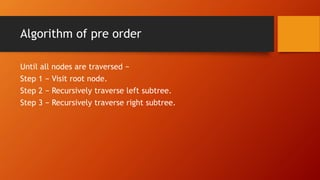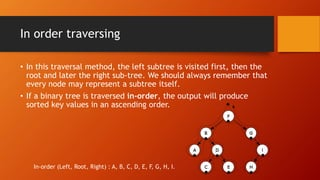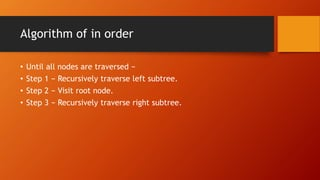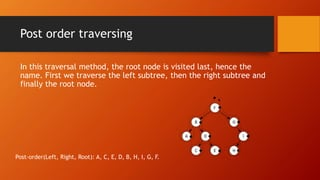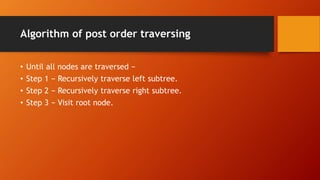1 von 26
Anzeige

### Binary search tree

1. Binary search tree Sana Yameen Presented By:
2. Definition • A binary search tree is a rooted binary tree, whose internal nodes each store a key (and optionally, an associated value) and each have two distinguished sub-trees, commonly denoted left and right. • The left sub-tree of a node has a key less than or equal to its parent node's key. • The right sub-tree of a node has a key greater than to its parent node's key.
3. Representation • BST is a collection of nodes arranged in a way where they maintain BST properties. Each node has a key and an associated value. While searching, the desired key is compared to the keys in BST and if found, the associated value is retrieved. • left_subtree (keys) ≤ node (key) ≤ right_subtree (keys)
4. Some BST terminologies • The root node is the top node of tree. • A child node has exactly one parent node and a parent node has at most two child nodes, sibling nodes shares the same parent node. • A leaf node has no child nodes, an interior node has at least one child node. • Every node in BST is a sub tree of the BST rooted at the node.
5. Basic Operations • Search • Inserting • Deleting • Traversing
6. Searching in BST • Searching a binary search tree for a specific key can be programmed recursively or iteratively. • To search a given key in Binary Search Tree, we first compare it with root, if the key is present at root, we return root. If key is greater than root’s key, we recur for right sub tree of root node. Otherwise we recur for left sub tree.
7. Algorithm of searching If root==search-data Return root Else While data not found If search-data>node-data Go to right sub tree Else Go to left sub tree If data found Return node End while Return data not found End if
8. Inserting Whenever an element is to be inserted, first locate its proper location. Start searching from the root node, then if the data is less than the key value, search for the empty location in the left subtree and insert the data. Otherwise, search for the empty location in the right sub tree and insert the data.
9. Binary search tree. Adding a value Adding a value to BST can be divided into two stages: • Search for a place to put a new element • Insert the new element to this place. • A new key is always inserted at leaf. We start searching a key from root till we hit a leaf node. Once a leaf node is found, the new node is added as a child of the leaf node.
10. Algorithm of inserting If root==null Create root node Return If root exist then If data> node Go to right sub tree Else Go to left sub tree End if Insert data End if
11. Deleting There are three possible cases to consider: • Deleting a node with no children: simply remove the node from the tree. • Deleting a node with one child: remove the node and replace it with its child. • Deleting a node with two children: call the node to be deleted N. Do not delete N. Instead, choose either its in-order successor node or its in-order predecessor node, R. Copy the value of R to N, then recursively call delete on the original R until reaching one of the first two cases.
12. Deleting a node with no children: simply remove the node from the tree.
13. Deleting a node with one child: remove the node and replace it with its child.
14. Deleting a node with two children:
15. Traversing Traversal of a binary tree is to access every node of binary tree at most. Such traversals are classified by the order in which the nodes are visited. There are three ways of traversing: Pre order (Root, Left, Right) In order (Left, Root, Right) Post order (Left, Right, Root)
16. Pre order traversing In this traversal method, the root node is visited first, then the left subtree and finally the right subtree. Pre-order (Root, Left, Right) : F, B, A, D, C, E, G, I, H.
17. Algorithm of pre order Until all nodes are traversed − Step 1 − Visit root node. Step 2 − Recursively traverse left subtree. Step 3 − Recursively traverse right subtree.
18. In order traversing • In this traversal method, the left subtree is visited first, then the root and later the right sub-tree. We should always remember that every node may represent a subtree itself. • If a binary tree is traversed in-order, the output will produce sorted key values in an ascending order. In-order (Left, Root, Right) : A, B, C, D, E, F, G, H, I.
19. Algorithm of in order • Until all nodes are traversed − • Step 1 − Recursively traverse left subtree. • Step 2 − Visit root node. • Step 3 − Recursively traverse right subtree.
20. Post order traversing In this traversal method, the root node is visited last, hence the name. First we traverse the left subtree, then the right subtree and finally the root node. Post-order(Left, Right, Root): A, C, E, D, B, H, I, G, F.
21. Algorithm of post order traversing • Until all nodes are traversed − • Step 1 − Recursively traverse left subtree. • Step 2 − Recursively traverse right subtree. • Step 3 − Visit root node.
22. Any Question
Anzeige